# How to Insert Square Root Symbol in Excel (5 Easy Ways)

Every software in the Microsoft Office package comes with its own purpose and application. Just like MS Word was built for word processing, MS Excel has been created to work on spreadsheets.

Both are different in their own ways. However, there does come a time when you might need to use some spreadsheets features in MS Word or some word processing features in MS Excel.

For example, symbols and special font features are not often used in spreadsheets, but there may be times when you might need it.

One such often used mathematical notation is that of the Square root.

Although the menu for this notation is not visible upfront in Microsoft Excel, there are a number of ways to insert a square root symbol in your Excel worksheet.

## Why We Might Need to use Square Root Symbol in MS Excel

Agreed that the square root symbol is not used as often as the function for finding the square root (the SQRT function).

However, you might want to maybe show values with the square root symbol and the square root of those values side by side.

Something as shown below (in the Standard Deviation column):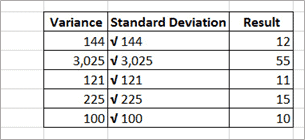Now let’s see how we can insert the square root symbol in Excel.

## Five Easy Ways to Insert the Square Root Symbol in MS Excel

Here are the easiest five ways in which you can insert the square root symbol in MS Excel.

### Use a Keyboard Shortcut

What easier way to insert a square root symbol than using a simple keyboard shortcut.

For this to work, you will need to use your numeric keypad though. In case you don’t have a numeric keypad (like may of the laptops these days), you can use any of the other methods covered later in this tutorial.

Below is the keyboard shortcut to insert a square root symbol in a cell in Excel:

`ALT + 251`

This means that you need to hold down your ALT key and press the numbers 2, 5, and 1 on the numeric keypad. Once you’re done, you will find the square root symbol inserted. However, note that this will not work if you try to use the numbers on the alphanumeric keypad of your keyboard.

Note: In some cases you may find that even with a numeric keypad, you don’t get the square root symbol when you use the above keyboard. This happens when your keyboard language is not set to English (United States). For this to work, you will have to change the keyboard input language (here are the steps on how to do this)

### Use the Symbol Feature

MS Office provides a really convenient way to insert commonly used symbols through its Symbol dialog box.

This feature is also available in MS Excel.

Here’s how you can use it to insert the Square Root symbol.

1. Click on the cell where you want to insert the symbol.
2. From the Insert Ribbon, select the Symbol option. This will cause the Symbol dialog box to appear.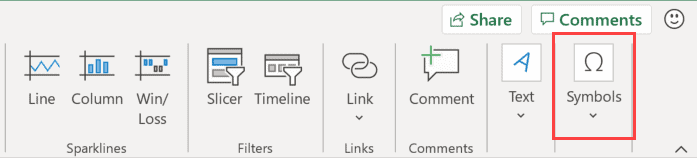3. Select Mathematical Operations from the Subset options drop-down. This will shorten the list of symbols to just those needed for mathematical operations, and you can easily find the square root symbol among these.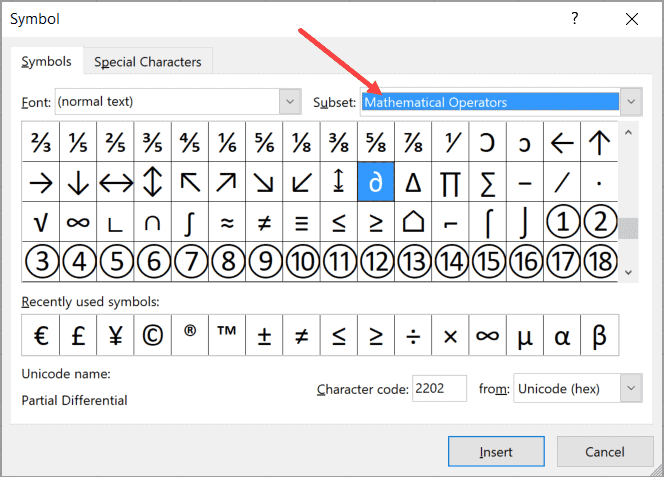4. Find the square root symbol in the list and double click on it. This will insert the square root symbol in the selected cell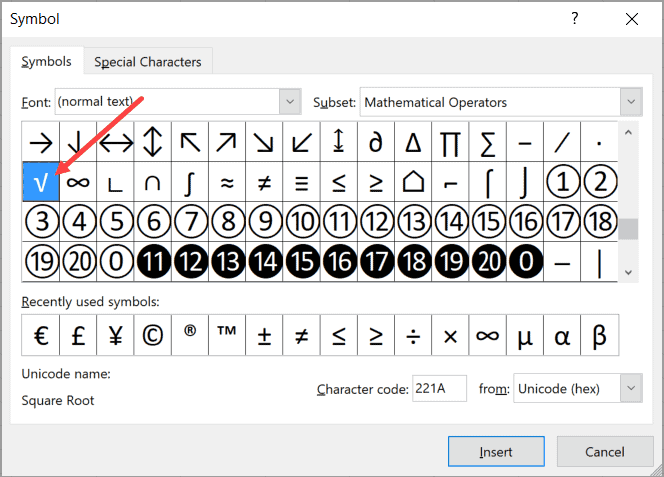Note that in case you have selected multiple cells in the worksheet, the symbol would be inserted in the active cell only.

Alternatively, you can also use the Character code to quickly find the symbol. Simply select Unicode(hex) in the from the drop-down and type 221A (as shown below).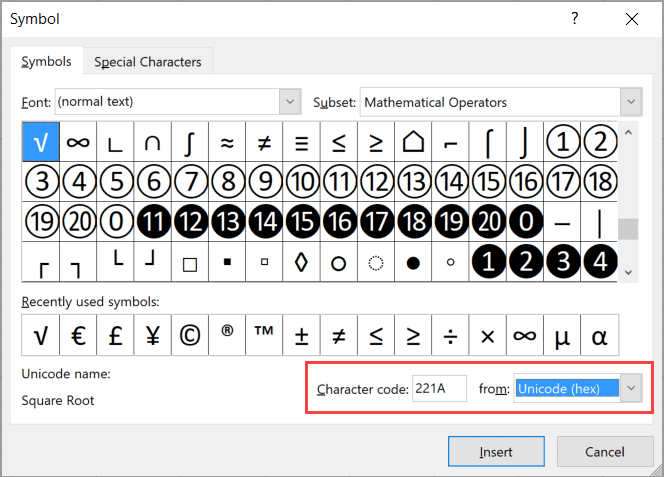Note that once you have inserted this symbol, it gets added to the Recently Used Symbols list at the bottom of the Symbols box. This will make it easier for you to use the symbol next time onwards as you will not need to search for it again.

### Use the UNICHAR Function

The UNICHAR() function is one of the in-built functions of MS Excel that gives the character value corresponding to a given decimal value.

There are numerical values (called ASCII codes) corresponding to each character, for example, the decimal value for the character ‘A’ is 67.

So if you type =UNICHAR(65) into a cell and hit the enter key, it will return A as the result of the formula.

In the same way, there is a numerical value corresponding to the square root function.

The value is 8730.

So to get the square root symbol in a cell, simply enter the following formula and hit the enter key

`=UNICHAR(8730)`

If you want to combine it with other formulas or cell references, you can easily do so. For example, to display it with the value in B3, you can type:

`=UNICHAR(8730)&B3`

Followed by the return key.

This method is quite useful when you have a column of values and want to add the square root to all these values in one go. In such cases all you need to do is select the format handle at the bottom right of the cell and drag it down.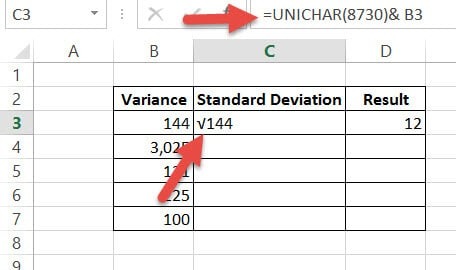### Just Copy-Paste

Instead of going through menus or trying to remember shortcuts, you can also just copy and paste the symbol below onto your spreadsheet, and then format and use it as needed.

`√`

In case you already have the square root symbol in the Excel workbook (or any MS Word or PowerPoint file), you can copy and paste from there as well.

### Change the Custom Number Format

When you insert a square root symbol into a cell (which may have a number after the symbol), the overall content of the cell becomes text. You can’t use this number in calculations as it’s now considered text by Excel.

If you want to show a square root symbol in a cell or range of cells but still want to keep the cell content as numbers, you can use the Custom Format method.

This method will show you the cell as having the square root symbol, but the actual value in the cell would continue to be the number (without the square root symbol).

Below are the steps to use Custom Number formatting to insert a square root symbol in a cell in Excel:

1. Right-click on the cell (or set of cells) where you want to insert the symbol.
2. Select Format Cells from the options that show up. This will open the Format Cells dialog box. (You can also use the keyboard shortcut Control +1)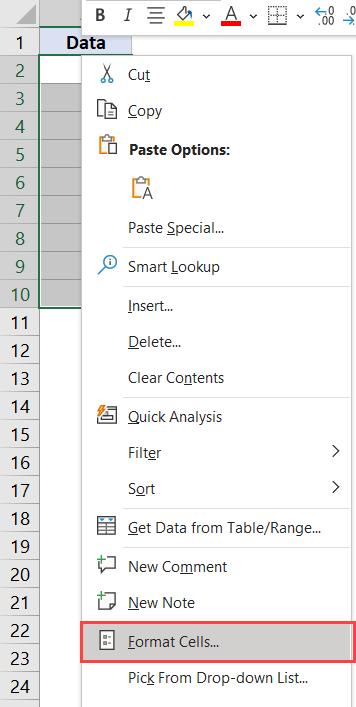3. From the Category pane, click on the Custom option. This will cause the Type field to appear on the right side.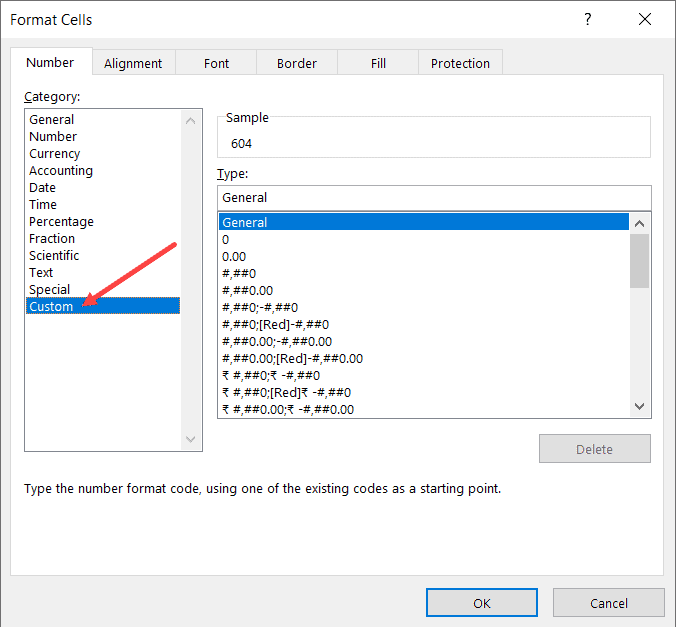4. In the Type field enter the below text: √General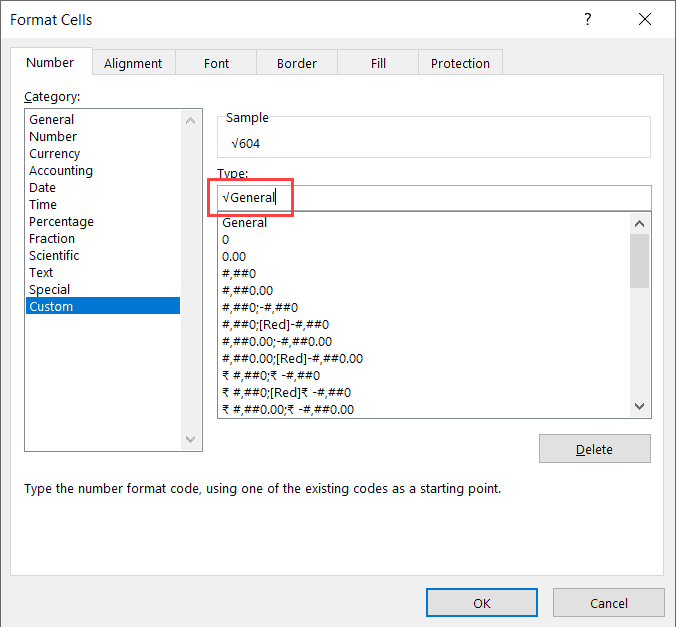5. Click OK.

You will find all the selected cells automatically formatted with the square root symbol.

To check if the actual value in the cell has changed or not, you can select any one of the cells and look at the formula bar. It should show you just the actual value of the cell, without the square root symbol.

There you go!

These are the 5 of the best ways to insert the square root symbol into your Excel spreadsheet.

I hope you found this Excel tutorial useful:

Other Excel tutorials you may like: